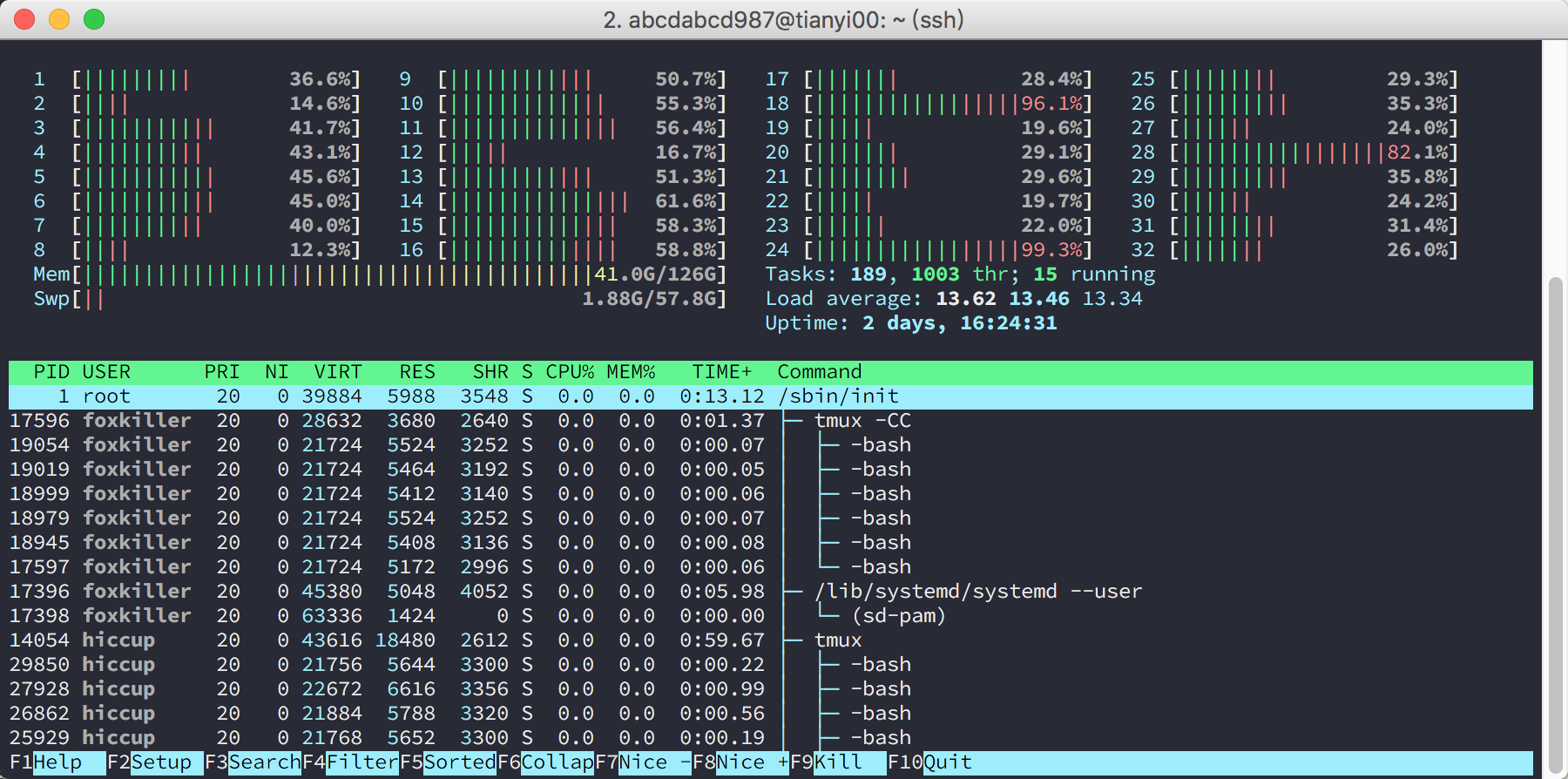# Python 多核并行计算

Nov 2, 2016 • python## multiprocessing vs threading

Python 自带的库又全又好用，这是我特别喜欢 Python 的原因之一。Python 里面有 multiprocessing和 threading 这两个用来实现并行的库。用线程应该是很自然的想法，毕竟（直觉上）开销小，还有共享内存的福利，而且在其他语言里面线程用的确实是非常频繁。然而，我可以很负责任的说，如果你用的是 CPython 实现，那么用了 threading 就等同于和并行计算说再见了（实际上，甚至会比单线程更慢），除非这是个IO密集型的任务。

## GIL

CPython 指的是 python.org 提供的 Python 实现。是的，Python 是一门语言，它有各种不同的实现，比如 PyPyJythonIronPython 等等……我们用的最多的就是 CPython，它几乎就和 Python 画上了等号。

CPython 的实现中，使用了 GIL 即全局锁，来简化解释器的实现，使得解释器每次只执行一个线程中的字节码。也就是说，除非是在等待IO操作，否则 CPython 的多线程就是彻底的谎言！

## multiprocessing.Pool

import multiprocessing

def f(x):
return x * x

cores = multiprocessing.cpu_count()
pool = multiprocessing.Pool(processes=cores)
xs = range(5)

# method 1: map
print pool.map(f, xs)  # prints [0, 1, 4, 9, 16]

# method 2: imap
for y in pool.imap(f, xs):
print y            # 0, 1, 4, 9, 16, respectively

# method 3: imap_unordered
for y in pool.imap_unordered(f, xs):
print(y)           # may be in any order


map 直接返回列表，而 i 开头的两个函数返回的是迭代器；imap_unordered 返回的是无序的。

cnt = 0
for _ in pool.imap_unordered(f, xs):
sys.stdout.write('done %d/%d\r' % (cnt, len(xs)))
cnt += 1


## 使用 Theano 或者 Tensorflow 等工具时的注意事项

could not retrieve CUDA device count: CUDA_ERROR_NOT_INITIALIZED


import multiprocessing

import tensorflow as tf
config = tf.ConfigProto()
config.gpu_options.allow_growth=True
sess = tf.Session(config=config)
while True:
res = sess.run(tf.constant('hello ' + name))
resultq.put(res)

if __name__ == '__main__':
resultq = multiprocessing.Queue()
p.start()

print(resultq.get())
print(resultq.get())

p.terminate()
p.join()


import multiprocessing

def init():
global tf
global sess
import tensorflow as tf
config = tf.ConfigProto()
config.gpu_options.allow_growth=True
sess = tf.Session(config=config)

def hello(name):
return sess.run(tf.constant('hello ' + name))

if __name__ == '__main__':
pool = multiprocessing.Pool(processes=2, initializer=init)
xs = ['world', 'abcdabcd987', 'Lequn Chen']
print pool.map(hello, xs)

Pool.apply_async：传入不确定参数

import multiprocessing as mp
import time

def foo_pool(x):
time.sleep(2)
return x*x

result_list = []
def log_result(result):
# This is called whenever foo_pool(i) returns a result.
# result_list is modified only by the main process, not the pool workers.
result_list.append(result)

def apply_async_with_callback():
pool = mp.Pool()
for i in range(10):
pool.apply_async(foo_pool, args = (i, ), callback = log_result)
pool.close()
pool.join()
print(result_list)

if __name__ == '__main__':
apply_async_with_callback()

©️2019 CSDN 皮肤主题: 编程工作室 设计师: CSDN官方博客